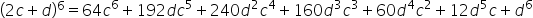Maths-
General
Easy

Question

# Use binomial theorem to expand (2c + d)6Hint:

## The correct answer is: values of the triangle

### Step 1 of 2:The given expression is (2c + d)6  , here x = 2c & y = d . The value of n=6, hence we would have 6+1=7 terms in the expression.Step 2 of 2:Substitute the values of (2c + d)6 in the binomial expressionThus, the expansion is:.

The expansion of the expression can also be found using the Pascal’s triangle. But, it is necessary to remember the values of the triangle to write down the expansion.#### With Turito Foundation.#### Get an Expert Advice From Turito.